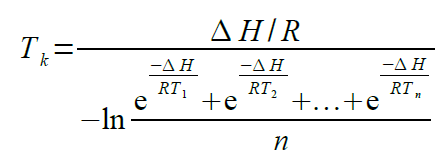# What is MKT?

Mean kinetic temperature (MKT) is defined by the ICH as “A single derived temperature that, if maintained over a defined period of time, affords the same thermal challenge to a drug substance or drug product as would be experienced over a range of both higher and lower temperatures for an equivalent defined period. The mean kinetic temperature is higher than the arithmetic mean temperature and takes into account the Arrhenius equation”.
Hence, the mean kinetic temperature may be considered as an isothermal storage temperature that simulates the non-isothermal effects of storage temperature variation. It is not a simple arithmetic mean but involves exponential and logarithmic relationships.
The Haynes formula can be used to calculate the MKT. It is higher than the arithmetic mean and takes into account the Arrhenius equation from which Haynes derived his formula. Thus, MKT is the single calculated temperature that stimulates the non-isothermal effects of storage temperature variations.• Tk = MKT in ºK
• ΔH = Heat of activation / activation energy
• R = Universal gas constant (8.3144? # 10-3 kJ.Mole-1 # ºK-1)
• Ti = temperature in ºK
• n = Total number of equal time periods over which data are collected

Mean kinetic temperature refers to a reference point, which can be calculated from a series of temperatures.
It differs from other means in that higher temperatures are given greater weight in computing the average.
This weighting is determined by a geometric transformation, the natural logarithm of the temperature number.
Disproportionate weighting of higher temperatures in a temperature series according to the MKT recognises the accelerated rate of thermal degradation of materials at these higher temperatures. MKT accommodates this non-linear effect of temperature.
It is calculated from the average storage temperatures recorded over a time period and a running average calculated from the average of weekly high and low temperatures. In order for MKT to be meaningful in anyway, an appropriate number of temperature / time sampling points should be used. MKT may only be applied in situations where temperature control of the storage area is good, but where occasional excursions do occur due to seasonal variation.
MKT may only be applied in cases where the scientific data regarding the thermal stability of the product in question, used to establish the original labelled storage conditions, permits limited excursions between 25ºC to 30ºC. The MA holder should be consulted as to whether these excursions affect the thermal stability of the products in question and hence whether or not the use of MKT is applicable.
Strict conditions should be applied to the use of MKT i.e.:

• It is only applicable to storage of products under controlled room temperature conditions (e.g. Those labelled “Do not store above 25ºC”)
• MKT is not appropriate for use for products requiring controlled low temperature storage.
• MKT cannot be used to compensate for poor temperature control of storage facilities due to e.g. poor design
• Excursions outside labelled storage requirements for products should be documented and investigated and reported to the MA holder
• Actual storage temperatures should not exceed 30ºC at any point if MKT is to be applied. i.e. for an MKT of 25ºC excursions between 15ºC and 30ºC are permitted.